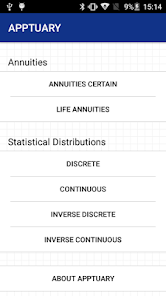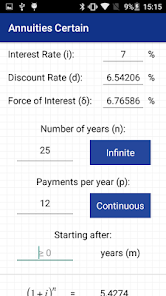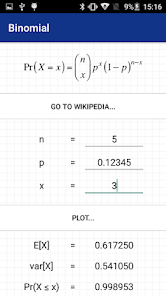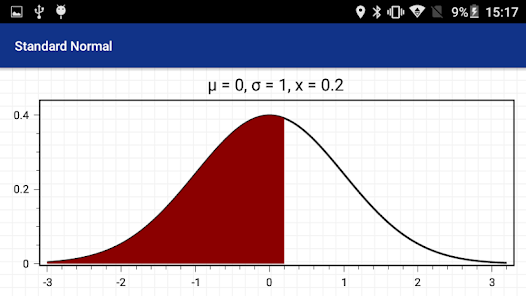# Apptuary: Actuarial Calculator

500+EveryoneApptuary is THE app to have if you're an Actuarial student or a qualified Actuary - PARTICULARLY if you're tired of looking up values in tables of figures.

Apptuary is the ONLY actuarial app that features downloadable Life Tables, and makes life-contingent annuity, compound-interest and statistical calculations fast and easy.

Apptuary can calculate:

• Values for life contingent annuities and assurances for single and joint lives, for any combination of interest rate and term.

• Values for annuities-certain for:
- any interest rate
- any number of payment years (including infinite)
- any number of payments per year (including continuous)
- any deferral period.

• Cumulative probabilities for a number of discrete and continuous statistical distributions, as well as values for the inverses of those cumulative distributions:
- Binomial
- Bernoulli
- Poisson
- Negative Binomial (Type I)
- Negative Binomial (Type II)
- Geometric
- Uniform (Discrete version)
- Standard Normal
- Normal (Gaussian)
- Exponential
- Gamma
- Chi-square
- Uniform (Continuous version)
- Lognormal
- Pareto (Two-parameter version)
- Weibull
- Burr

• The means and variances for the above distributions PLUS the following:
- Beta
- Pareto (Three parameter version)
Updated on
Apr 2, 2017

## Data safety